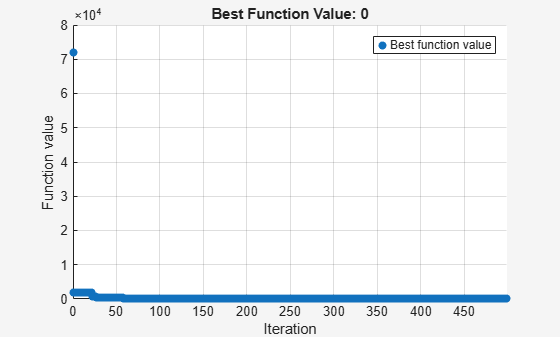# Mixed-Integer Surrogate Optimization

This example shows how to solve an optimization problem that involves integer variables. Beginning in R2019b, `surrogateopt` accepts integer constraints. In this example, find the point `x` that minimizes the `multirosenbrock` function over integer-valued arguments ranging from –3 to 6 in ten dimensions. The `multirosenbrock` function is a poorly scaled function that is difficult to optimize. Its minimum value is 0, which is attained at the point `[1,1,...,1]`. Code for the `multirosenbrock` function appears at the end of this example.

```rng(1,'twister') % For reproducibility nvar = 10; % Any even number lb = -3*ones(1,nvar); ub = 6*ones(1,nvar); fun = @multirosenbrock; intcon = 1:nvar; % All integer variables [sol,fval] = surrogateopt(fun,lb,ub,intcon)``````surrogateopt stopped because it exceeded the function evaluation limit set by 'options.MaxFunctionEvaluations'. ```
```sol = 1×10 1 1 1 1 1 1 1 1 1 1 ```
```fval = 0 ```

In this case, `surrogateopt` finds the solution.

### Helper Function

This code creates the `multirosenbrock` helper function.

```function F = multirosenbrock(x) % This function is a multidimensional generalization of Rosenbrock's % function. It operates in a vectorized manner, assuming that x is a matrix % whose rows are the individuals. % Copyright 2014 by The MathWorks, Inc. N = size(x,2); % assumes x is a row vector or 2-D matrix if mod(N,2) % if N is odd error('Input rows must have an even number of elements') end odds = 1:2:N-1; evens = 2:2:N; F = zeros(size(x)); F(:,odds) = 1-x(:,odds); F(:,evens) = 10*(x(:,evens)-x(:,odds).^2); F = sum(F.^2,2); end```